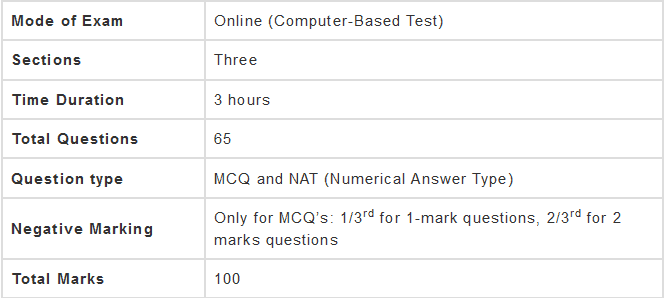# Syllabus - Electrical Engineering, GATE - Practice Notes - GATE

## GATE: Syllabus - Electrical Engineering, GATE - Practice Notes - GATE

The document Syllabus - Electrical Engineering, GATE - Practice Notes - GATE is a part of GATE category.
All you need of GATE at this link: GATE

Before getting into the details of GATE syllabus for EEE, let’s have an overview of GATE exam pattern for EEE.The three sections of the GATE EE paper are:
(i) General Aptitude
(ii) Engineering Mathematics
(iii) Subject-Specific Section

Let us look into the detailed GATE syllabus for EEE for each of these sections.

Syllabus for General Aptitude (GA) (Common to all Papers)
• Verbal Ability: English grammar, sentence completion, verbal analogies, word groups, instructions, critical reasoning and verbal deduction.
• Numerical Ability: Numerical computation, numerical estimation, numerical reasoning and data interpretation.
Electrical Engineering

1. Engineering Mathematics

• Linear Algebra: Matrix Algebra, Systems of linear equations, Eigenvalues, Eigenvectors.
• Calculus: Mean value theorems, Theorems of integral calculus, Evaluation of definite and improper integrals, Partial Derivatives, Maxima and minima, Multiple integrals, Fourier series, Vector identities, Directional derivatives, Line integral, Surface integral, Volume integral, Stokes’s theorem, Gauss’s theorem, Green’s theorem.
• Differential equations: First order equations (linear and nonlinear), Higher order linear differential equations with constant coefficients, Method of variation of parameters, Cauchy’s equation, Euler’s equation, Initial and boundary value problems, Partial Differential Equations, Method of separation of variables.
• Complex variables: Analytic functions, Cauchy’s integral theorem, Cauchy’s integral formula, Taylor series, Laurent series, Residue theorem, Solution integrals.
• Probability and Statistics: Sampling theorems, Conditional probability, Mean, Median, Mode, Standard Deviation, Random variables, Discrete and Continuous distributions, Poisson distribution, Normal distribution, Binomial distribution, Correlation analysis, Regression analysis.
• Numerical Methods: Solutions of nonlinear algebraic equations, Single and Multi‐step methods for differential equations.
• Transform Theory: Fourier Transform, Laplace Transform, z‐Transform.

2. Electric Circuits

• Network graph, KCL, KVL, Node and Mesh analysis.
• Transient response of dc and ac networks, Sinusoidal steady‐state analysis.
• Resonance, Passive filters, Ideal current and voltage sources, The venin’s theorem.
• Norton’s theorem, Superposition theorem, Maximum power transfer theorem.
• Two‐port networks, Three-phase circuits, Power and power factor in ac circuits.

3. Electromagnetic Fields

• Coulomb's Law, Electric Field Intensity, Electric Flux Density, Gauss's Law, Divergence, Electric field and potential due to point, line, plane and spherical charge distributions, Effect of dielectric medium, Capacitance of simple configurations.
• Biot‐Savart’s law, Ampere’s law, Curl, Faraday’s law, Lorentz force, Inductance, Magnetomotive force, Reluctance.
• Magnetic circuits, Self and Mutual inductance of simple configurations.

4. Signals and Systems

• Representation of continuous and discrete‐time signals, Shifting and scaling operations.
• Linear Time-Invariant and Causal systems, Fourier series representation of continuous periodic signals, Sampling theorem.
• Applications of Fourier Transform, Laplace Transform and z-Transform.

5. Electrical Machines

• Single-phase transformer: equivalent circuit, phasor diagram, open circuit and short circuit tests, regulation and efficiency.
• Three-phase transformers: connections, parallel operation; Auto‐transformer, Electromechanical energy conversion principles.
• DC machines: separately excited, series and shunt, motoring and generating mode of operation and their characteristics, starting and speed control of dc motors.
• Three-phase induction motors: Principle of operation, types, performance, torque-speed characteristics, no-load and blocked rotor tests, equivalent circuit, starting and speed control; Operating principle of single-phase induction motors.
• Synchronous machines: cylindrical and salient pole machines, performance, regulation and parallel operation of generators, starting of synchronous motor, characteristics; Types of losses and efficiency calculations of electric machines.

6. Power Systems

• Power generation concepts, ac and dc transmission concepts, Models and performance of transmission lines and cables, Series and shunt compensation.
• Electric field distribution and insulators, Distribution systems, Per‐unit quantities, Bus admittance matrix, Gauss-Seidel and Newton-Raphson load flow methods.
• Voltage and Frequency Control, Power factor correction, Symmetrical components, Symmetrical and unsymmetrical fault analysis, Principles of overcurrent, differential and distance protection.
• Circuit breakers, System stability concepts, Equal area criterion.

7. Control Systems

• Mathematical modelling and representation of systems, Feedback principle, transfer function, Block diagrams and signal flow graphs.
• Transient and Steady‐state analysis of linear time-invariant systems, Routh-Hurwitz and Nyquist criteria, Bode plots, Root loci, Stability analysis, Lag, Lead and Lead‐Lag compensators.
• P, PI and PID controllers, State-space model, State transition matrix.

8. Electrical and Electronic Measurements

• Bridges and Potentiometers, Measurement of voltage, current, power, energy and power factor.
• Instrument transformers, Digital voltmeters and multimeters, Phase, Time and Frequency measurement.
• Oscilloscopes, Error analysis.

9. Analog and Digital Electronics

• Characteristics of diodes, BJT, MOSFET; Simple diode circuits: clipping, clamping, rectifiers.
• Amplifiers: Biasing, Equivalent circuit and Frequency response; Oscillators and Feedback amplifiers; Operational amplifiers: Characteristics and applications.
• Simple active filters, VCOs and Timers, Combinational and Sequential logic circuits, Multiplexer, De-multiplexer, Schmitt trigger, Sample and hold circuits, A/D and D/A converters.
• 8085Microprocessor: Architecture, Programming and Interfacing.

10. Power Electronics

• Characteristics of semiconductor power devices- Diode, Thyristor, Triac, GTO, MOSFET, IGBT.
• DC to DC conversion: Buck, Boost and Buck-Boost converters.
• Single and three-phase configuration of uncontrolled rectifiers, Line commutated thyristor-based converters, Bidirectional ac to dc voltage source converters, Issues of line current harmonics.
• Power factor, Distortion factor of ac to dc converters, Single-phase and three-phase inverters, Sinusoidal pulse width modulation.
The document Syllabus - Electrical Engineering, GATE - Practice Notes - GATE is a part of GATE category.
All you need of GATE at this link: GATEUse Code STAYHOME200 and get INR 200 additional OFF

Track your progress, build streaks, highlight & save important lessons and more!

,

,

,

,

,

,

,

,

,

,

,

,

,

,

,

,

,

,

,

,

,

,

,

,

;